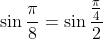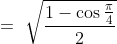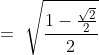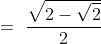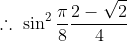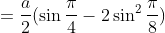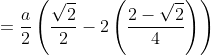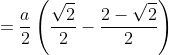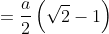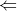1.   If is a factor of , Find the values of and
Solution
Let  .
Since is a factor of , the remainder when is divided by is 0.
By polynomial long division we can find the remainder.

Hence we have

and .
Similarly, we can say ,
When , and
When , .
-------------------------------------------------------------------------
2.   If is divisible by , prove that .
Solution
Since is divisible by , The remainder when is divided by = is zero.
By polynomial long division,

and .
.
(a)    Let be a triangle with right angle and hypotenuse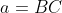.
(See the figure.)
If the inscribed circle touches the hypotenuse at D,
show that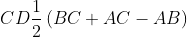.
(b)   If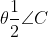, express the radius of the inscribed circle in terms  of and
(c)   If  is fixed and  varies, find the maximum value of .
Solution
Let   be the centre of the circle and and be points of
tangency of and respectively.(given)
Draw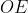and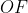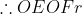Since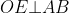and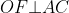,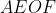is a square.
Therefore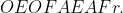Let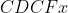.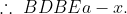(a)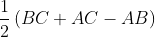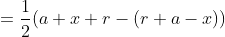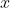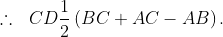(b)   Draw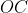. Since is incentre,bisects.
In right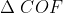,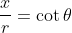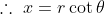In right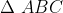, By Pythagoras Theorem,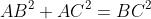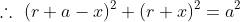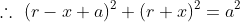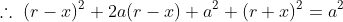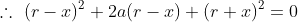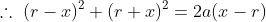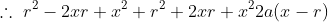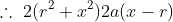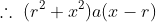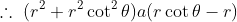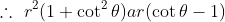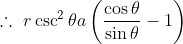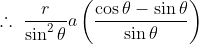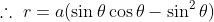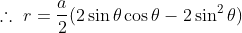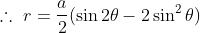(c)   Since is fixed and  varies,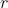is a function of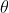and the rate of
change ofwith respect tois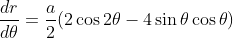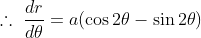has stationary value when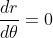.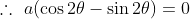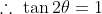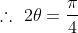and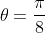.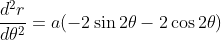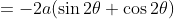When,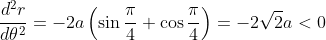.will be maximum value when.